#### Parameter Initialization

An important stage when you prepare for the model fitting process is initialization of the model parameters. As stated earlier, nonlinear optimization techniques are used in the fitting process. These techniques assist in the estimation of the model parameters, and being nonlinear means they can be very sensitive to selection of the initial values.

You can specify initial values close to the expected estimates when you have a relatively simple problem, such as in the example of the section Getting Started: VARIOGRAM Procedure. In the case of nested models the selection of initial values can be more challenging because you have to assess the level of contribution for each one of the nested components.

The VARIOGRAM procedure features automatic selection of initial values based on the recommendations in Jian, Olea, and Yu (1996). Specifically, if you compute the estimated empirical semivariogram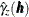at k lags, then:

• The default initial nugget effect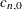is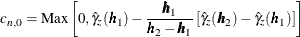• The default initial slope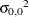and initial exponent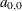for the power model are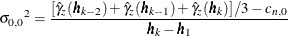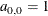• The default initial scaleand initial rangefor all other models are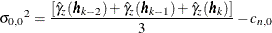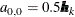When you use the Matérn form, PROC VARIOGRAM sets the default initial value for the Matérn smoothness to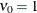.

These rules are observed in the case of single, non-nested model fitting, and they are slightly modified to apply for nested model fitting as follows: Assume that you want to fit a nested model composed of m structures. As stated in the section Nested Models, the nugget effect is a single parameter and is independent of the number of nested structures in a model. Also, the sum of the nested structure scales and the nugget effect, if any, must be equal to the total variance. For this reason, PROC VARIOGRAM simply divides the initial scale value it would assign to a non-nested model into m components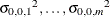. For the range parameter, the VARIOGRAM procedure sets the initial range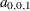of the first nested structure equal to the value it would assign to a non-nested model initial range. Then, the initial range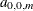of the m-component is set recursively to half the value of the initial range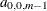of the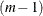-component.

Your empirical semivariogram must have nonmissing estimates at least at three lags so that you can use the automated fitting feature in PROC VARIOGRAM. Overall, if you specify a model form with q parameters to fit to an empirical semivariogram with nonmissing estimates at k lags, then the fitting problem is well-defined only when the degrees of freedom are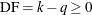.

A potential numerical issue is that fitting could momentarily lead the fitting parameters to near-zero semivariance values at lags away from zero distance. The theoretical semivariance is always positive for any distance larger than zero, and this is also a requirement for the numerical computation of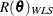in weighted least squares fitting. Such numerical issues are unlikely but possible, depending on the data set you use and the parameter initial values. If an event of nonpositive semivariance at a given lag occurs during an iteration, then PROC VARIOGRAM transparently adds a minimal amount of variance at that lag for the specific iteration. You can control this amount of variance with the NEPSILON= option of the MODEL statement. It is recommended that you leave this parameter at its default value.

The section concludes with a reminder of the fitting process sensitivity to the initial parameter values selection. The VARIOGRAM procedure facilitates this selection for you by using the simple rules shown earlier. However, the suggested initial values might not always be the best choice. In simple cases, such as the introductory example in the section Getting Started: VARIOGRAM Procedure, this approach is very convenient and effective.

In principle, you are strongly encouraged to experiment with initial values. You want to make sure that the fitting process leads the model parameters to converge to estimates that make sense for your problem. When a parameter estimate seems unreasonable on the basis of your problem specification (for example, a model scale might be estimated to be 10 times the size of your sample variance, or the estimate of a range might be zero), PROC VARIOGRAM produces a note to let you know about a potentially ambiguous fit. These issues are examined in more detail in the section Quality of Fit.